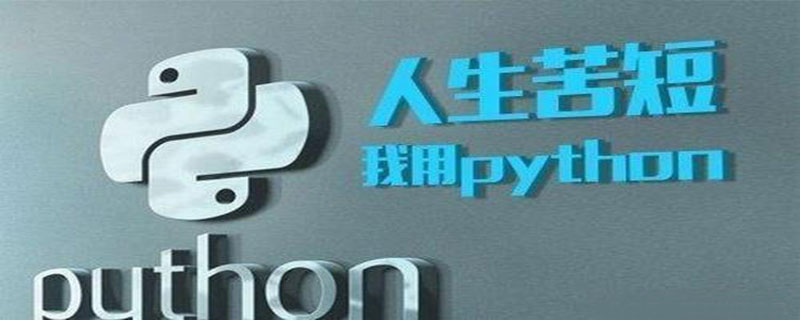## 值得一看！使用Python变量时要避免的3个错误

339次阅读Python编程中经常遇到一些莫名其妙的错误， 其实这不是语言本身的问题， 而是我们忽略了语言本身的一些特性导致的，今天就来看下使用Python变量时导致的3个不可思议的错误， 以后在编程中要多多注意。

1、 可变数据类型作为函数定义中的默认参数

```def search_for_links(page, add_to=[]):

```def fn(var1, var2=[]):
var2.append(var1)
print(var2)
fn(3)
fn(4)
fn(5)```

```

```

```
[3,4]
[3,4,5]```

```fn(3,)
[4,3]```

```def fn(var1, var2=None):
ifnot var2:
var2 =[]
var2.append(var1)```

2、 可变数据类型作为类变量

```class URLCatcher(object):
urls =[]
self.urls.append(url)```

```a =URLCatcher()
b =URLCatcher()
print(b.urls)
print(a.urls)```

```['http://www.google.com','http://www.pythontab.com']

```class URLCatcher(object):
def __init__(self):
self.urls =[]
self.urls.append(url)```

3、 可变的分配错误

`a ={'1':"one",'2':'two'}`

```b = a
b['3']='three'```

`{'1':"one",'2':'two','3':'three'}`

`{'1':"one",'2':'two','3':'three'}`

```c =(2,3)
d = c
d =(4,5)```

`b = a[:]`

`b = a.copy()`